# 216CHAPTER 3 Differentiation Rules35-38 Find y" by implicit differentiation.fi35. x² + 4y2 = 436. x² + xy + y² = 3ti37. sin y + cos x = 138. x- y' = 749-6039. If xy + e = e, find the value of y" at the point wherepossiblX = 0.49. y40. If x2 + xy + y'= 1, find the value of y" at the point51. y =where x = 1.53. F(CAS 41. Fanciful shapes can be created by using the implicit plottingcapabilities of computer algebra systems.(a) Graph the curve with equation54. y =27

Question
7 views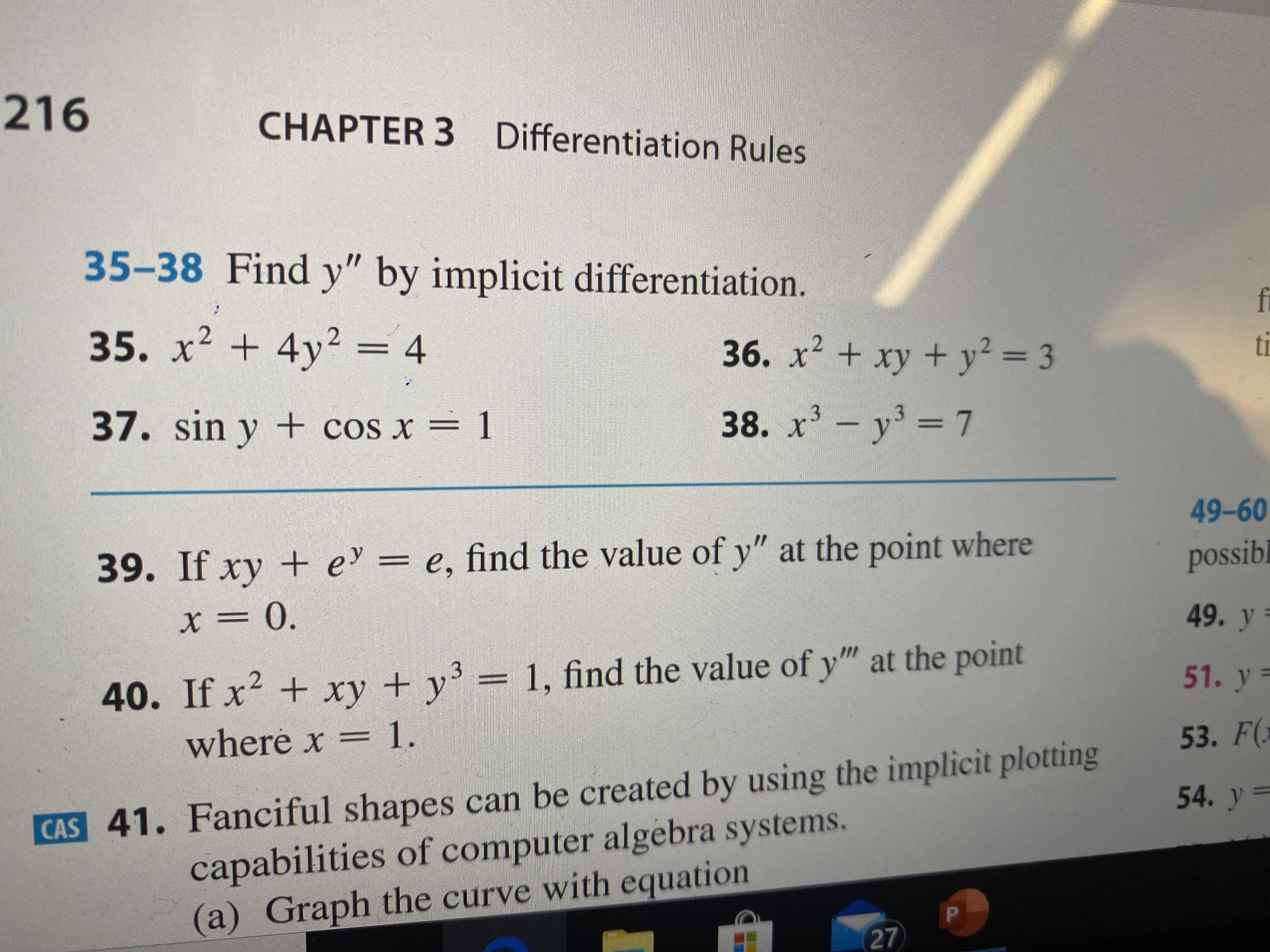help_outlineImage Transcriptionclose216 CHAPTER 3 Differentiation Rules 35-38 Find y" by implicit differentiation. fi 35. x² + 4y2 = 4 36. x² + xy + y² = 3 ti 37. sin y + cos x = 1 38. x- y' = 7 49-60 39. If xy + e = e, find the value of y" at the point where possibl X = 0. 49. y 40. If x2 + xy + y'= 1, find the value of y" at the point 51. y = where x = 1. 53. F( CAS 41. Fanciful shapes can be created by using the implicit plotting capabilities of computer algebra systems. (a) Graph the curve with equation 54. y = 27 fullscreen
check_circle

Step 1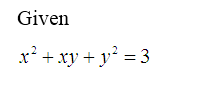Step 2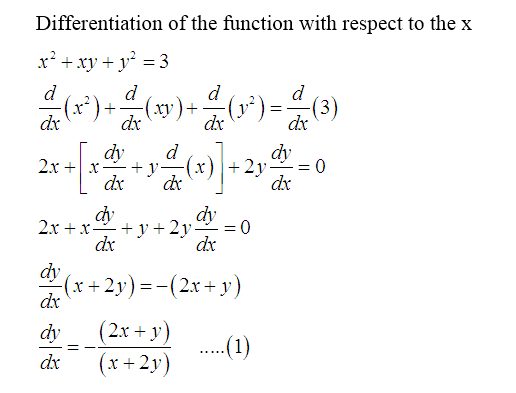Step 3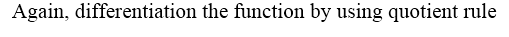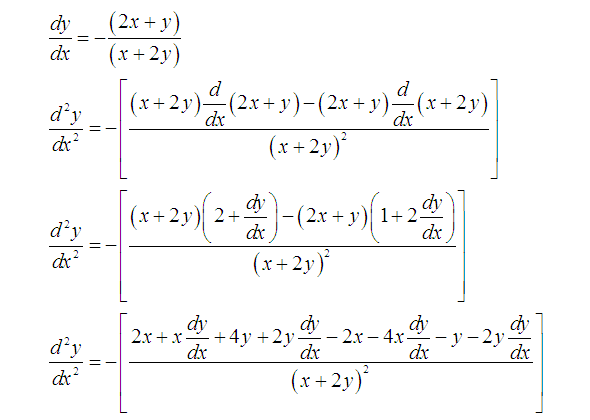...

### Want to see the full answer?

See Solution

#### Want to see this answer and more?

Solutions are written by subject experts who are available 24/7. Questions are typically answered within 1 hour.*

See Solution
*Response times may vary by subject and question.
Tagged in